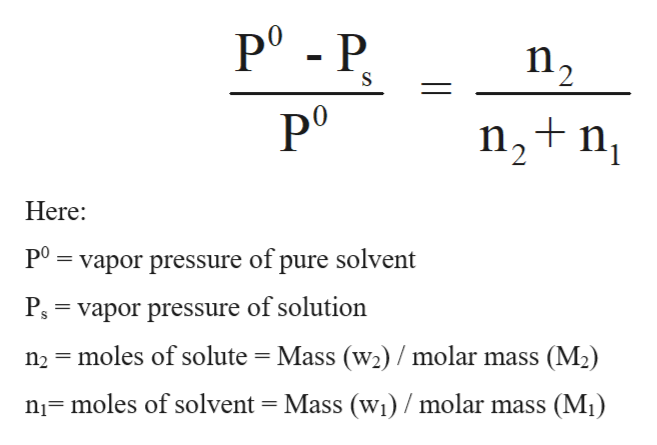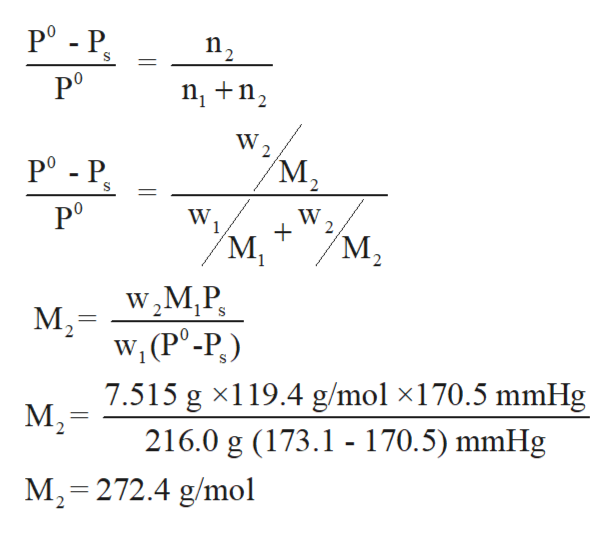# he common laboratory solvent chloroform is often used to purify substances dissolved in it. The vapor pressure of chloroform, CHCl3, is 173.1 mm Hg at 25 °C. In a laboratory experiment, students synthesized a new compound and found that when 7.515 grams of the compound were dissolved in 216.0 grams of chloroform, the vapor pressure of the solution was 170.5 mm Hg. The compound was also found to be nonvolatile and a nonelectrolyte. What is the molecular weight of this compound? chloroform = CHCl3 = 119.4 g/molAnswer:MW =____  g/mol

Question
158 views

he common laboratory solvent chloroform is often used to purify substances dissolved in it. The vapor pressure of chloroform, CHCl3, is 173.1 mm Hg at 25 °C.

In a laboratory experiment, students synthesized a new compound and found that when 7.515 grams of the compound were dissolved in 216.0 grams of chloroform, the vapor pressure of the solution was 170.5 mm Hg. The compound was also found to be nonvolatile and a nonelectrolyte.

What is the molecular weight of this compound?

chloroform = CHCl3 = 119.4 g/mol
MW =____  g/mol

check_circle

Step 1

Relative lowering in the vapor pressure of a solution is a colligative property that depends on the number of particles present in the solution. Some other examples of colligative properties are osmotic pressure, elevation in boiling point, depression in freezing point etc.

Step 2

The relative lowering in the vapor pressure can be formulated on the basis of Raoult's law.

Raoult's law states that the vapor pressure of a component at a given temperature is the product of the mole fraction of that component and the vapor pressure of that component in the pure state.help_outlineImage TranscriptionclosePo - P n2 n2+n1 Here P0 vapor pressure of pure solvent P = vapor pressure of solution n2 moles of solute = Mass (w2)/molar mass (M2) n1 moles of solvent = Mass (w1) / molar mass (M) fullscreen
Step 3

P0 = vapor pressure of pure solvent = 173.1 mmHg

Ps = vapor pressure of solution = 170.5 mmHg

Mass (w2) = 7.515 g

Mass (w1) = 216.0 g

Molar mass (M1) = 119.4 g/mol

Molar mass (...help_outlineImage TranscriptionclosePo - P n2 S nn W. Po - P M2 Po W W 2 M2 M, M W2M,P w, (P°-P,) 7.515 g x119.4 g/mol x170.5 mmHg M2= 216.0 g (173.1 - 170.5) mmHg M2-272.4 g/mol fullscreen

### Want to see the full answer?

See Solution

#### Want to see this answer and more?

Solutions are written by subject experts who are available 24/7. Questions are typically answered within 1 hour.*

See Solution
*Response times may vary by subject and question.
Tagged in

### Solutions